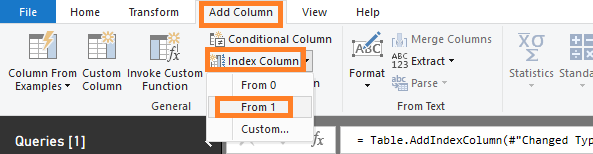cancel
Showing results for
Did you mean:Helper I

## Display Current row value in another row in different column

Dear All,

I am a bit new to Dax, I have a requirement to display the current row value(Sum of Duration) in the next row of another column(Sum of Duration1).

Description is a column of Table

Sum of Duration is a Measure

Rank_Measure is a measure

Rank Next is a Measure

Sum of Duration1 is a measure that I am trying to create.

 Description(Column-Part of Table) Sum of Duration(Measure) Rank_Measures(Measure) Rank Next(Measure) Sum of Duration1(Measure) Component1 10,94 6 7 Component2 9,00 7 8 10,94 Component3 8,00 8 9 9,00 Component4 7,00 9 10 8,00 Component5 6,60 10 11 7,00 Component6 5,40 11 12 6,60 Component7 3,20 12 13 5,40

1 ACCEPTED SOLUTIONCommunity Support

Hi @Amir851 ,

You may remain your [Rank_Measure], try to create another Rank column, then create measure [Sum of Duration1] as follows:

``````Column: Rank = RANKX(ALL(Table1),[Sum of Duration],,DESC, Dense)

Measure: Sum of Duration1 = CALCULATE(Table1[Sum of Duration]),FILTER(ALLSELECTED(Table1), Table1[Rank] =MAX(Table1[Rank])-1))``````

Best Regards,

Amy

Community Support Team _ Amy

If this post helps, then please consider Accept it as the solution to help the other members find it more quickly.

3 REPLIES 3Community Support

Hi @Amir851 ,

You may remain your [Rank_Measure], try to create another Rank column, then create measure [Sum of Duration1] as follows:

``````Column: Rank = RANKX(ALL(Table1),[Sum of Duration],,DESC, Dense)

Measure: Sum of Duration1 = CALCULATE(Table1[Sum of Duration]),FILTER(ALLSELECTED(Table1), Table1[Rank] =MAX(Table1[Rank])-1))``````

Best Regards,

Amy

Community Support Team _ Amy

If this post helps, then please consider Accept it as the solution to help the other members find it more quickly.Community Support

Hi @Amir851 ,

You can create measure like DAX below.

Sum of Duration1 = CALCULATE(Table1[Sum of Duration]),FILTER(ALLSELECTED(Table1), Table1[Rank_Measure] =Table1[Rank_Measure]-1))

Or you may add Index column to original table in Query Editor.Then create measure like DAX below.

Sum of Duration1 = CALCULATE(Table1[Sum of Duration]),FILTER(ALLSELECTED(Table1), Table1[Index] =MAX(Table1[Index])-1))

Best Regards,

Amy

Community Support Team _ Amy

If this post helps, then please consider Accept it as the solution to help the other members find it more quickly.Helper I

Dear @v-xicai ,

I have tried that Dax which you mentioned earlier itself but it was not working and it returned empty value, The issue is that I cannot create an index column in this situation because Rank_Measure is a measure and it has four slicer acting upon it.is there is any other way to do it.

Thanks

Amir